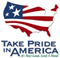U.S. GEOLOGICAL SURVEY

Water-Resources Investigations Report 02-4298

# Development of Regression Equations to Estimate Flow Durations and Low-Flow-Frequency Statistics in New Hampshire Streams

## ABSTRACT

Regression equations and basin-characteristic digital datasets were developed to help water-resource managers estimate surface-water resources during periods of low flow in New Hampshire. The regression equations were developed to estimate statistics for the seasonal and annual low-flow-frequency and seasonal period-of-record and period-of-record flow durations. Because streamflow is maintained by ground-water discharge during periods of low flow, these equations also will aid in the assessment of ground-water availability. Ultimately, the equations and datasets developed herein can be combined with data on water withdrawals, discharges, and interbasin transfers in a geographic information system (GIS) to allow assessments of water use and water availability in any drainage basin in the State of New Hampshire.

Regression equations developed in this study provide estimates of the seasonal (spring, summer, fall, and winter) and annual 7-day 2-year (7Q2) and 7-day 10-year (7Q10) low-flow-frequency values, as well as seasonal period-of-record and period-of-record flow durations (60-, 70-, 80-, 90-, 95-, and 98-percent exceedences) for ungaged reaches of unregulated New Hampshire streams. Regression equations were developed using seasonal and annual low-flow statistics from 58 to 60 continuous-record stream-gaging stations in New Hampshire and nearby areas in neighboring states, and measurements of various characteristics of the drainage basins that contribute flow to those stations.

The estimating equations for the seasonal and annual 7Q2 and 7Q10 values were developed using generalized-least-squares (GLS) regression analyses. T he GLS equations developed for these flow statistics gave average prediction errors that ranged from 11 to 61 percent.

The estimating equations for flow-duration exceedence frequency values were developed using ordinary-least-squares (OLS) regression analysis. The OLS equations developed for these flow statistics gave average prediction errors ranging from 14 to 79 percent.

A total of 93 measurable drainage-basin characteristics were selected as possible predictor variables. Of these 93 variables, the following 10 were determined to be statistically significant predictors for at least one of the dependent variables: drainage area, average basin slope, maximum basin elevation, average summer gage precipitation for 1961-90, average spring gage precipitation for 1961-90, average mean annual basin temperature for 1961-90, average mean summer basin temperature for 1961-90, average winter basin-centroid precipitation for 1961-90, percent of the basin that is coniferous, and percent of the basin that is mixed coniferous and deciduous. These 10 basin characteristics were selected because they were statistically significant based on several statistical parameters that evaluated which combination of characteristics contributed the most to the predictive accuracy of the regression-equation models. A GIS is required to measure the values of the predictor variables for the equations developed in this study.

Abstract

Introduction

Purpose and scope

Previous Studies

Description of Study Area

Acknowledgments

Low-Flow Frequency and Flow-Duration Estimating Methods at Stream-Gaging Stations

Streamflow Database

Flow-Duration Statistics

Low-Flow-Frequency Statistics

Low-Flow-Frequency and Flow-Duration Estimating Methods at Ungaged Stream Sites

Drainage-Area Ratio Approach

Concurrent-Flow Approach

Regression-Equation Approach

Development of Regression Model for Estimation of Low-Flow-Frequency and Flow-Duration Statistics

Drainage-Basin Characteristics

Regression Analysis

Regression-Equation Development

Prediction Interval

Computation Example

Physical Basis for Regression Relations

Limitations on the Use of Regression Equations

Summary and Conclusions

Selected References

Appendix 1. Basin Characteristics Tested for Significance in the Regression Analysis

Appendix 2. Flow-Duration Statistics Estimated Using Available Data and Regression Equation Predicted Values for the Period-of-Record

Appendix 3. Flow-Duration Statistics Estimated Using Available Data and Regression Equation Predicted Values for the Winter Season, January 1 to March 15

Appendix 4. Flow-Duration Statistics Estimated Using Available Data and Regression Equation Predicted Values for the Spring Season, March 16 to May 31

Appendix 5. Flow-Duration Statistics Estimated Using Available Data and Regression Equation Predicted Values for the Summer Season, June 1 to October 31

Appendix 6. Flow-Duration Statistics Estimated Using Available Data and Regression Equation Predicted Values for the Fall Season, November 1 to December 31

Appendix 7. Low-Flow Statistics Estimated Using Available Data and Regression Equation Predicted Values for Annual and Seasonal Periods

View the full report in PDF (11.0 MB)

Document Accessibility:  Adobe Systems Incorporated has information about PDFs and the visually impaired. This information provides tools to help make PDF files accessible. These tools and the accessible reader may be obtained free from Adobe at Adobe Access.

For further information contact

Keith Robinson, Director

New Hampshire-Vermont Water Science Center

361 Commerce Way

Pembroke, NH 03275

(603) 226-7800

dc_nh@usgs.gov

 AccessibilityFOIAPrivacyPolicies and Notices U.S. Department of the Interior, U.S. Geological Survey Persistent URL: Page Contact Information: Contact USGS Last modified: Wednesday, February 22 2006, 01:36:57 PM